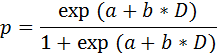SORTIE-ND
Software for spatially-explicit simulation of forest dynamics

# Logistic bi-level mortality

This behavior calculates the probability of survival according to a logistic equation, with the possibility of two sets of parameters for each species: one for high-light conditions and one for low-light conditions. This can also be used alone without the light levels.

Trees killed by this behavior will have a mortality reason code of "natural".

### Parameters for this behavior

Parameter nameDescription
Logistic Bi-Level - Low-Light "a"The "a" parameter used in low-light conditions.
Logistic Bi-Level - Low-Light "b"The "b" parameter used in low-light conditions.
Logistic Bi-Level - High-Light "a"The "a" parameter used in high-light conditions.
Logistic Bi-Level - High-Light "b"The "b" parameter used in high-light conditions.
Logistic Bi-Level - High-Light Mortality Threshold (0-100)The threshold between low-light and high-light parameters, as a value between 0 and 100.

### How it works

The equation used by this behavior to calculate survival probability is:where

• p - annual probability of survival
• a - in high-light conditions, this is the Logistic Bi-Level - High-Light "a" parameter; in low-light conditions, this is the Logistic Bi-Level - Low-Light "a" parameter
• b - in high-light conditions, this is the Logistic Bi-Level - High-Light "b" parameter; in low-light conditions, this is the Logistic Bi-Level - Low-Light "b" parameter
• D - tree diam, in cm; diam10 for seedlings, DBH for others

If the timestep length is not one year, the actual probability of survival for the timestep is calculated as p' = p T, where p is the annual probability of survival, p' is the timestep probability of survival, and T is the number of years per timestep. Once the survival probability for the timestep is known for a tree, then a random number is compared to this probability to determine if the tree lives or dies.

Light levels come from the Storm Light grid produced by the Storm Light behavior. The threshold between the use of high-light and low-light parameters is set in the Logistic Bi-Level - High-Light Mortality Threshold (0-100) parameter.

This behavior can also be used without Storm Light. In this case, only the low-light mortality parameters are used.

### How to apply it

This behavior can be applied to seedlings, saplings, and adults of any species. If you wish to use the light-level parameter switch, also use the Storm Light behavior.